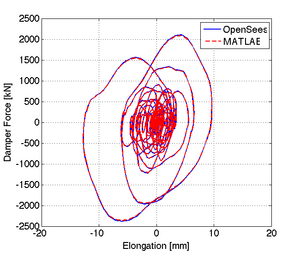# Calibration of Maxwell Material

Jump to navigationJump to search

Example posted by: Dr. Dimitrios G. Lignos (McGill University)

This example demonstrates how to conduct a calibration of a viscous damper using the maxwell model.

The files needed to analyze this structure in OpenSees are included here:

Supporting procedure files

All files are available in a compressed format here: Calibration_Maxwell_example.zip

The rest of this example describes the model and shows the analysis results.

## Model Description

The viscous damper is modeled with the Force-Based Beam-Column element. This element follow a Maxwell hysteretic response. An idealized schematic of the model is presented in Figure 1.

The units of the model are mm, N, and seconds.

### Basic Geometry

The basic geometry of the viscous damper is defined by input variables for the length L=5000mm, and area A = 12000mm2. Two nodes are used for the geometry of the damper.

### Damper Section

A square section is used to define the area A of the damper (Adamper = 12000.0mm2).

### Damper Links

Force-Based Beam-Column elements are used to link the two nodes that define the geometry of the viscous damper with n=5 sections of integration and the damper section defined previously.

### Constraints

Node 1 is pinned. A roller is assigned to node 2

### Maxwell Material

To model the viscous damper the Maxwell is used. The input parameters that are selected for the damper example are as follows: Axial Stiffness K = 500000.0 N/mm, Viscous Coefficient C=100000.0 N(s/mm)0.30 and exponent a=0.30.

### Loading

The viscous damper is loaded with a displacement history that is called Damper.txt in the axial loading direction. A linear pattern is selected and the node of application is Node 2.

### Recorders

The recorders used in this example include:

### Analysis

In order to calibrate the viscous damper a multiple support excitation option is selected with application of displacement as the imposed motion. This is necessary since an integration step dt should be specified for integration of strain histories for each time step.

## Results

### Comparison of OpenSees Model with MATLAB based Maxwell modelFigure 2. Viscous Damper Response and Comparison With MATLAB based Script

The force - displacement relationship from the maxwell damper are shown in Figure 2. In order to obtain the displacement of the damper we multiply the strain in section 3 of the damper times the length L=5000mm. A comparison of the response with a MATLAB based script is also shown in the same figure. Results are identical.